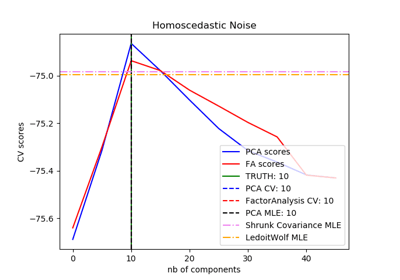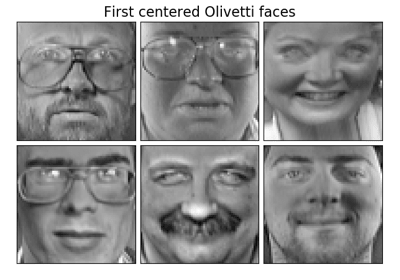/scikit-learn

# sklearn.decomposition.FactorAnalysis

`class sklearn.decomposition.FactorAnalysis(n_components=None, tol=0.01, copy=True, max_iter=1000, noise_variance_init=None, svd_method=’randomized’, iterated_power=3, random_state=0)` [source]

Factor Analysis (FA)

A simple linear generative model with Gaussian latent variables.

The observations are assumed to be caused by a linear transformation of lower dimensional latent factors and added Gaussian noise. Without loss of generality the factors are distributed according to a Gaussian with zero mean and unit covariance. The noise is also zero mean and has an arbitrary diagonal covariance matrix.

If we would restrict the model further, by assuming that the Gaussian noise is even isotropic (all diagonal entries are the same) we would obtain `PPCA`.

FactorAnalysis performs a maximum likelihood estimate of the so-called `loading` matrix, the transformation of the latent variables to the observed ones, using expectation-maximization (EM).

Read more in the User Guide.

Parameters: `n_components : int | None` Dimensionality of latent space, the number of components of `X` that are obtained after `transform`. If None, n_components is set to the number of features. `tol : float` Stopping tolerance for EM algorithm. `copy : bool` Whether to make a copy of X. If `False`, the input X gets overwritten during fitting. `max_iter : int` Maximum number of iterations. `noise_variance_init : None | array, shape=(n_features,)` The initial guess of the noise variance for each feature. If None, it defaults to np.ones(n_features) `svd_method : {‘lapack’, ‘randomized’}` Which SVD method to use. If ‘lapack’ use standard SVD from scipy.linalg, if ‘randomized’ use fast `randomized_svd` function. Defaults to ‘randomized’. For most applications ‘randomized’ will be sufficiently precise while providing significant speed gains. Accuracy can also be improved by setting higher values for `iterated_power`. If this is not sufficient, for maximum precision you should choose ‘lapack’. `iterated_power : int, optional` Number of iterations for the power method. 3 by default. Only used if `svd_method` equals ‘randomized’ `random_state : int, RandomState instance or None, optional (default=0)` If int, random_state is the seed used by the random number generator; If RandomState instance, random_state is the random number generator; If None, the random number generator is the RandomState instance used by `np.random`. Only used when `svd_method` equals ‘randomized’. `components_ : array, [n_components, n_features]` Components with maximum variance. `loglike_ : list, [n_iterations]` The log likelihood at each iteration. `noise_variance_ : array, shape=(n_features,)` The estimated noise variance for each feature. `n_iter_ : int` Number of iterations run.

`PCA`
Principal component analysis is also a latent linear variable model which however assumes equal noise variance for each feature. This extra assumption makes probabilistic PCA faster as it can be computed in closed form.
`FastICA`
Independent component analysis, a latent variable model with non-Gaussian latent variables.

#### Examples

```>>> from sklearn.datasets import load_digits
>>> from sklearn.decomposition import FactorAnalysis
>>> transformer = FactorAnalysis(n_components=7, random_state=0)
>>> X_transformed = transformer.fit_transform(X)
>>> X_transformed.shape
(1797, 7)
```

#### Methods

 `fit`(X[, y]) Fit the FactorAnalysis model to X using EM `fit_transform`(X[, y]) Fit to data, then transform it. `get_covariance`() Compute data covariance with the FactorAnalysis model. `get_params`([deep]) Get parameters for this estimator. `get_precision`() Compute data precision matrix with the FactorAnalysis model. `score`(X[, y]) Compute the average log-likelihood of the samples `score_samples`(X) Compute the log-likelihood of each sample `set_params`(**params) Set the parameters of this estimator. `transform`(X) Apply dimensionality reduction to X using the model.
`__init__(n_components=None, tol=0.01, copy=True, max_iter=1000, noise_variance_init=None, svd_method=’randomized’, iterated_power=3, random_state=0)` [source]
`fit(X, y=None)` [source]

Fit the FactorAnalysis model to X using EM

Parameters: `X : array-like, shape (n_samples, n_features)` Training data. `y : Ignored` self
`fit_transform(X, y=None, **fit_params)` [source]

Fit to data, then transform it.

Fits transformer to X and y with optional parameters fit_params and returns a transformed version of X.

Parameters: `X : numpy array of shape [n_samples, n_features]` Training set. `y : numpy array of shape [n_samples]` Target values. `X_new : numpy array of shape [n_samples, n_features_new]` Transformed array.
`get_covariance()` [source]

Compute data covariance with the FactorAnalysis model.

`cov = components_.T * components_ + diag(noise_variance)`

Returns: `cov : array, shape (n_features, n_features)` Estimated covariance of data.
`get_params(deep=True)` [source]

Get parameters for this estimator.

Parameters: `deep : boolean, optional` If True, will return the parameters for this estimator and contained subobjects that are estimators. `params : mapping of string to any` Parameter names mapped to their values.
`get_precision()` [source]

Compute data precision matrix with the FactorAnalysis model.

Returns: `precision : array, shape (n_features, n_features)` Estimated precision of data.
`score(X, y=None)` [source]

Compute the average log-likelihood of the samples

Parameters: `X : array, shape (n_samples, n_features)` The data `y : Ignored` `ll : float` Average log-likelihood of the samples under the current model
`score_samples(X)` [source]

Compute the log-likelihood of each sample

Parameters: `X : array, shape (n_samples, n_features)` The data `ll : array, shape (n_samples,)` Log-likelihood of each sample under the current model
`set_params(**params)` [source]

Set the parameters of this estimator.

The method works on simple estimators as well as on nested objects (such as pipelines). The latter have parameters of the form `<component>__<parameter>` so that it’s possible to update each component of a nested object.

Returns: self
`transform(X)` [source]

Apply dimensionality reduction to X using the model.

Compute the expected mean of the latent variables. See Barber, 21.2.33 (or Bishop, 12.66).

Parameters: `X : array-like, shape (n_samples, n_features)` Training data. `X_new : array-like, shape (n_samples, n_components)` The latent variables of X.

## Examples using `sklearn.decomposition.FactorAnalysis`Model selection with Probabilistic PCA and Factor Analysis (FA)Faces dataset decompositions